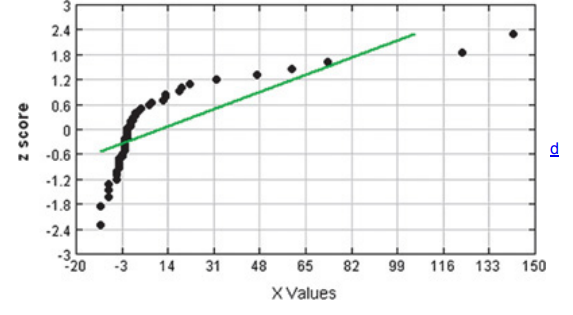×
Get Full Access to Elementary Statistics - 12 Edition - Chapter 6.6 - Problem 8bsc
Get Full Access to Elementary Statistics - 12 Edition - Chapter 6.6 - Problem 8bsc

×

# Solution: Interpreting Normal Quantité Plots. In Exercises,ISBN: 9780321836960 18

## Solution for problem 8BSC Chapter 6.6

Elementary Statistics | 12th Edition

• Textbook Solutions
• 2901 Step-by-step solutions solved by professors and subject experts
• Get 24/7 help from StudySoup virtual teaching assistantsElementary Statistics | 12th Edition

4 5 1 347 Reviews
26
1
Problem 8BSC

Interpreting Normal Quantile Plots. In Exercises 5–8, examine the normal quantile plot and determine whether the sample data appear to be from a population with a normal distribution.

Flight Departure Delays The normal quantile plot represents flight departure delay times (minutes) from Data Set 15 in Appendix B.Step-by-Step Solution:

Step 1 of 1 :

Given, The normal quantile plot represents flight departure delay times (minutes) from Data Set 15.The claim is to determine whether the sample data appear to be from a population with a normal distribution.

Conclusion :

Not normal. The points are not reasonably close to a straight line pattern, and there appears to be a pattern that is not a straight line pattern.

Step 2 of 1

##### ISBN: 9780321836960

This full solution covers the following key subjects: normal, plot, data, Flight, Departure. This expansive textbook survival guide covers 121 chapters, and 3629 solutions. The full step-by-step solution to problem: 8BSC from chapter: 6.6 was answered by , our top Statistics solution expert on 03/15/17, 10:30PM. The answer to “?Interpreting Normal Quantile Plots. In Exercises 5–8, examine the normal quantile plot and determine whether the sample data appear to be from a population with a normal distribution.Flight Departure Delays The normal quantile plot represents flight departure delay times (minutes) from Data Set 15 in Appendix B.” is broken down into a number of easy to follow steps, and 47 words. Since the solution to 8BSC from 6.6 chapter was answered, more than 313 students have viewed the full step-by-step answer. Elementary Statistics was written by and is associated to the ISBN: 9780321836960. This textbook survival guide was created for the textbook: Elementary Statistics, edition: 12.

Unlock Textbook Solution